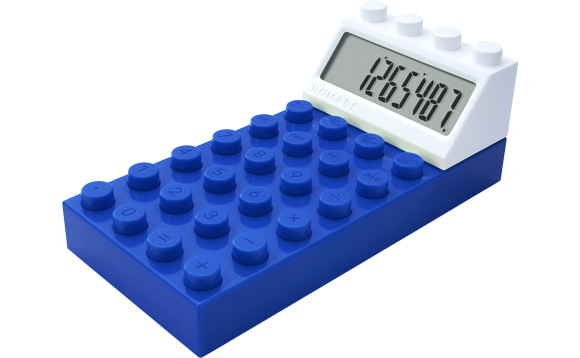# Calculator FunOnce, I was playing with my calculator at school during the break. I was doing many things with the number 16, when I decided to calculate the square root of it. The result, of course, was 4. However, I pressed, by accident, the square root button again, and the result that was being shown was 2. I then thought of what had just happened: I had a number, then I calculated the square root of it, and the square root of the result was an integer, which was not a perfect square. In other words, the result of the square root of a perfect square (16) was also a perfect square (4). Let the initial number be $x$, and the perfect square results be $x_{1}, x_{2}, x_{3}, ... ,x_{m}$, where $x_{m}$ is the last possible result, which is not a perfect square. As an explicit example, I used $x = 16$ that day at school, so $m$ was $2$ and $x_{m} = 2$. Consider that $n$ and $k$ are positive integers. In order to maximize the value of $m$, $x$ should be of the form:

Note: the exponent of $x_m$ must be minimized.

×